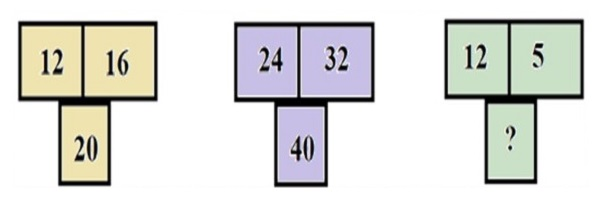# Question of the day-Logical Reasoning

2019-06-18 | Team PendulumEdu

Q. The numbers in the blocks given below follows a certain pattern. Study the pattern and choose the number which will replace the question mark?A. 23

B. 15

C. 13

D. 16

Solution

Observing the numbers in the first block we get the pattern as

$$12^2 + 16^2 = 20^2$$

Observing the numbers in the first block we get the pattern as

$$24^2 +32^2 = 40^2$$

Thus,

$$12^2 + 5^2 = 13^2$$

Hence, (C) is the required solution.0